## 1 Introduction

Motivation Nowadays, more than half of all Internet traffic is encrypted according to a 2017 EFF report , with Google reporting that 95% of its traffic is encrypted as of October 2020 . This trend has also been facilitated by efforts like the free digital certificate issuer Let’s Encrypt servicing 87 million active (unexpired) certificates and 150 million unique domains at the end of 2018 .

This widespread Internet traffic encryption is enabled by protocols that allow two parties (where one or both parties have a public key certificate) to establish a secure communication channel over the insecure Internet. Typically, the parties first authenticate all parties holding a public key certificate and agree on a session key—the key exchange phase. Then, this session key is used to encrypt the communication during the session—the secure channel phase. We will refer to such protocols as secure channel establishment protocols.

The main secure channel establishment protocol in use today is Transport Layer Security (TLS). The session key establishment of the widely deployed standard TLS 1.2  requires 2 round-trip times (RTTs) of end-to-end communication for a full connection and 1 RTT for resumption (which saves 1 RTT), before sending encrypted data. TLS 1.2 typically runs on top of TCP  for reliable transport, which adds another 1 RTT of establishing a TCP connection before the TLS connection. Further, this TCP cost is paid every time the two parties communicate with each other, even if the connection is interrupted and then immediately resumed. Given that most encrypted traffic is web traffic, this cost represents a significant performance bottleneck, a nuisance to users, and financial loss to companies. For instance, back in 2006 Amazon found that every 100ms of latency cost them 1% in sales , while a typical RTT on a connection from New York to London is 70ms .

Not surprisingly, many efforts in recent years have focused on reducing latency in secure channel establishment protocols. The focus has been on reducing the number of interactions (or RTTs) during session establishment and resumption without sacrificing much security. The most important protocols addressing this goal are TLS 1.3 , Google’s Quick UDP Internet Connections (QUIC) [20, 46, 62], and the new design for QUIC  (which we refer to as QUIC[TLS]  to indicate that it borrows the key exchange from TLS 1.3).

With TLS 1.3, it is possible to achieve 0-RTT resumption, i.e., the client is able to send encrypted 0-RTT data on the first flight to the server with session resumption. This is achieved by utilizing a session ticket that was saved during a previous communication and multiple keys (which we call stage keys) that can be set within one session, of which some keys are set faster (with slightly less security) so that data can be encrypted earlier. However, for the most common case where TLS 1.3 runs over TCP, the client still has to wait 1 RTT before transmitting any encrypted data due to the aforementioned TCP connection. One optimization for TCP, called TCP Fast Open (TFO) [17, 59], extends TCP to allow for 0-RTT resumption connections, so that the client may begin data transmission immediately. The mechanism underlying this optimization is a cookie saved from previous communication, similar to the ticket used by TLS 1.3. As a result, TLS 1.3 over TFO offers 0-RTT resumption.

Like TLS 1.3, QUIC uses weaker initial keys, under which data can be encrypted earlier, and a token saved from previous communication between the parties. However, unlike TLS, QUIC operates over the very simple UDP  rather than TCP. Instead of relying on TCP for reliability, flow control, and congestion control, QUIC implements its own data transmission functionality, integrating connection establishment with key exchange. These features allow QUIC to have 1-RTT full connections and 0-RTT resumption connections.

In Table 1, we show the cost of establishing full and resumption connections for several layered protocol options achieving end-to-end security. These include TLS 1.2 over TCP, TLS 1.3 over TCP, TLS 1.3 over TFO, QUIC over UDP, and QUIC[TLS] over UDP. It is clear that the last three win in terms of the number of interactions. But how does their security compare?

Related Work At first glance, the question is easy to answer. Recent works have done formal security analyses of TLS 1.3 [10, 11, 14, 18, 19, 21, 24,25,26,27, 42, 43, 47] and Google’s QUIC [29, 49]. Most works confirm that (the cryptographic cores of) both protocols are provably secure under reasonable computational assumptions. Moreover, as shown in [27, 49], their 0-RTT data transmission designs cannot achieve the same strong security guaranteed by classical key exchange protocols with at least one RTT. In particular, for TLS 1.3 and QUIC, their 0-RTT keys do not provide forward secrecy and the 0-RTT data suffers from replay attacks. (Note that it is possible to construct key exchange protocols to provide both forward secrecy for 0-RTT keys and replay resistance for 0-RTT data, but the existing constructions [4, 23, 33] require either large server-side storage or computationally expensive public-key operations.) Overall, it might seem that all three layered protocols mentioned above are equally secure.

However, a closer look reveals that the answer is not that simple. First, all aforementioned formal security analyses, except for  analyzing QUIC’s IP-spoofing protection (also known as address validation), did not consider packet-level availability attacks, i.e., those targeting availability properties like reliable delivery of messages (via packets). Therefore, it is not clear at the packet level what security can be achieved and what attacks can be prevented by these protocols. In other words, we have no formal understanding of what security can be obtained when layering protocols. Also, TFO uses some cryptographic primitives, such as a cookie, to prevent IP spoofing, but no formal analysis has been done. Furthermore, the security of QUIC[TLS] has not been formally analyzed (although some security aspects can be reduced to those of Google’s QUIC and TLS 1.3).

Our Contributions To compare security, we first need to define a general protocol syntax for secure channel establishment and fix a security model for it. Since the only provable security analysis that studies security related to packet-level availability attacks is , we take their quick connections (QC) protocol syntax and the associated quick authenticated and confidential channel establishment (QACCE) security model as our starting point.

Protocol definition. To accommodate protocol syntaxes of TLS 1.3 and QUIC[TLS], we extend the QC protocol to a more general multi-stage authenticated and confidential channel establishment (msACCE) protocol, which allows more keys to be established during each session. Note that QC is a two-stage extension (i.e., two keys established within one session) to the ACCE protocol syntax proposed by Jager et al. , while our msACCE further extends QC to capture multiple keys.

Security definitions. We extend QC’s associated QACCE security model  to two msACCE security models that are general enough for all layered secure channel establishment protocols listed in Table 1. Our first model is fairly standard and for core cryptographic security goals Server Authentication and Channel Security, where the former guarantees the client authenticates its intended server’s identity upon accepting the final session key and the latter ensures data confidentiality and integrity. Our second model is novel and for packet-level security beyond those captured by the standard goals, which we illustrate below.

First, we follow QACCE  to consider IP-spoofing security and further extend it to our stronger IP-Spoofing Prevention notion that additionally captures IP-spoofing attacks in the full connections.

Next, we design several novel notions for packet integrity. We first define header integrity to capture the integrity of the entire unencrypted packet header. (Note that previous models like QACCE only cover the header integrity implied by the authenticity security of the underlying authenticated encryption scheme.) To enable fine-grained security analyses and comparisons, we split the above notion into two related ones, Key Exchange (KE) Header Integrity and Secure Channel (SC) Header Integrity, which capture header integrity during the key exchange phase and secure channel phase, respectively. Furthermore, we define the notion of KE Payload Integrity to cover availability attacks that modify the payloads of key exchange packets. We note that unlike the availability attacks shown in , successful attacks under the above new notions do not affect the client’s session key establishment and therefore are harder or impossible to detect by the client.

Finally, we formalize the new goal of Reset Authentication to deal with attacks that forge a reset packet to abruptly terminate (without completing) an honest party’s session, i.e., it ensures that a session should be reset only by the intended party who lost its state (e.g., due to server reboots). Such stateless reset security may be confused with secure termination proposed by . However, secure termination targets a very different goal: it ensures that upon receipt of a termination message the receiver has received all messages that were sent and will ever be sent by the sender, i.e., it ensures a session is completed as expected. As modeled in , this property can be reduced to the security of the underlying channel protocol, which in our case is guaranteed by Channel Security (and hence not captured by our second model). Note that all termination message(s) are encrypted and authenticated in the established secure channel, while a reset packet used for a stateless reset typically cannot be protected because the issuing party has already lost all session states including the keying material (Table 2).

Security analyses. Equipped with our new models, we study the security and availability properties provided by TFO+TLS 1.3, UDP+QUIC, and UDP+QUIC[TLS], with results summarized below.

We first confirm that all protocols provably satisfy the standard security notions of Server Authentication and Channel Security given that their building blocks are secure. The results mostly follow from prior works and we just have to argue that they still hold for the extended model. Similarly, prior results showed that QUIC is secure against IP spoofing, which can be extended to our stronger notion for both UDP+QUIC and UDP+QUIC[TLS]. As for TFO+TLS 1.3, its IP-Spoofing Prevention security relies on TCP sequence number randomization and TFO’s cookie mechanism (but no prior former analysis confirmed its security). We prove that TFO+TLS 1.3 does satisfy this security assuming that the underlying TFO cookie generation function is a pseudorandom function.

Regarding SC Header Integrity, we show that while UDP+QUIC and UDP+QUIC[TLS] are secure, TFO+TLS 1.3, on the other hand, is insecure because it allows header-only packets to be sent in the secure channel phases and does not authenticate the TCP headers of encrypted packets. This theoretical result captures practical availability attacks that the networking community has been slowly uncovering via manual investigation over the last 30 years [3, 16, 31, 32, 38,39,40, 44, 45, 52, 57, 58, 63, 70], such as TCP flow control manipulation, TCP acknowledgment injection, etc.

We next show that none of the three protocols satisfies KE Header Integrity. For TFO+TLS 1.3, this result leads to a TFO cookie removal attack that we discover, which allows the attacker to undermine the whole benefit of TFO without being detected by the communicating parties. Then, we show that UDP+QUIC is not secure in the sense of KE Payload Integrity. This leads to a new availability attack that we call ServerReject Triggering. Note that unlike the QUIC attacks (e.g., server config replay attack, connection ID manipulation attack, etc.) discovered in , ServerReject Triggering is harder to detect. Similar attacks also work for UDP+QUIC[TLS]. We show that TFO+TLS 1.3, on the other hand, achieves KE Payload Integrity.

We further show that neither TFO+TLS 1.3 nor UDP+QUIC provides Reset Authentication, justifying the TCP Reset attack  relevant for TFO+TLS 1.3 and the PublicReset attack for UDP+QUIC. On the other hand, we prove that UDP+QUIC[TLS] does guarantee Reset Authentication with its new stateless reset feature, by relying on the unpredictability of the reset tokens.

We note that the new UDP+QUIC[TLS] protocol achieves the strongest overall security of the three designs. While formally it does not provide KE Payload Integrity, the related attacks can also happen in TFO+TLS 1.3 in a similar way; the latter satisfies KE Payload Integrity mainly because its availability functionalities are all carried in its protocol headers rather than payloads. More importantly, UDP+QUIC[TLS] is the only protocol that guarantees Reset Authentication.

Even though QUIC[TLS] may not be able to sustain the competition in the long run despite stronger security, we hope our models will help protocol designers and practitioners better understand the important security aspects of existing and new secure channel establishment protocols.

Paper Organization The rest is organized as follows. We provide an overview of relevant design information for TFO, TLS 1.3, QUIC, and QUIC[TLS] in Sect. 2. Section 3 specifies our notations and preliminaries. Our msACCE protocol and its security are formally defined in Sects. 4 and  5 presents the details of our security analyses. Section 6 concludes our paper.

## 2 Background

Network protocols are designed and implemented following a layered network stack model where each layer has its own functionality, defines an interface for use by higher layers, and relies only on the properties of lower layers. In this work, we are concerned with three layers: network, represented by the IP protocol; transport, represented by UDP, TCP, or TFO; and application, represented by TLS, QUIC, or QUIC[TLS].

### 2.1 TLS 1.3 over TFO

TCP Fast Open TFO is an optimization for the TCP protocol. TCP itself provides the following services to an application (or higher protocol): (1) reliability, (2) ordered delivery, (3) flow control, and (4) congestion control. It is connection-oriented and consists of three phases: connection establishment, data transfer, and connection tear-down. TCP relies on control information from its header to implement this functionality. For example, as shown in Fig. 1, control bits specify what type of packets are sent over the network, which determines whether the packets are establishing a new connection, sending data, acknowledging data, or tearing down the connection.

The disadvantage of layering protocols is that higher level protocols have no control over the internal mechanics of lower level protocols and can interact with them only through defined interfaces. A protocol using standard TCP for transferring data needs to wait for connection establishment at the TCP layer to complete before it receives notification of a new connection and can begin its own processing and data transfer. A standard TCP connection is established with a three-way handshake, which consists of three packets SYN, SYN-ACK, ACK as shown in Fig. 2. SYN carries a random sequence number that is acknowledged by SYN-ACK, and SYN-ACK carries another random sequence number that is acknowledged by ACK.

The TFO optimization introduces a simple modification to the TCP connection establishment handshake to reduce the 1-RTT connection establishment latency of TCP and allow for 0-RTT handshakes, so that data transmission may begin immediately. TFO fulfills the same design goals mentioned for TCP above, assuming the connection is established correctly.

The mechanism through which 0-RTT is achieved is a cookie that is obtained by the client the first time it communicates with a server and cached for later uses. This cookie is intended to prevent replay attacks while avoiding the need for servers to keep expensive state. It is generated by the server, authenticates client IP address, and has a limited lifetime. Generation and verification have low overhead.

Cookies are sent in the TFO option field in SYN packets. The first two message exchanges in Fig. 2a show how a cookie is obtained. The client requests a cookie by using the TFO option in the SYN with the cookie field set to empty (nil), indicating that it would like to use TFO. The server generates an appropriate cookie and places it in the TFO option field of the SYN-ACK. The client caches this cookie for subsequent connections to this server. If a cookie was not provided, the client instead caches the negative response, indicating that TFO connections should not be tried to this server, for some time.

In subsequent connections to this server (first message in Fig. 2b), the client places its cached TFO cookie in the TFO option in the SYN packet. The client is also allowed to send 0-RTT data in the remainder of the SYN packet. This might be an HTTP GET request or a TLS ClientHello message. When the server receives the SYN, it will validate the cookie. If the cookie is valid, it responds with a SYN-ACK acknowledging the 0-RTT data and a response to the 0-RTT data. If the cookie is invalid (expired or otherwise), a full handshake is required and any initial data is ignored.

TLS 1.3 TLS 1.3 provides confidentiality, authentication, and integrity of communication over a secure channel between a client and a server. This is accomplished in two phases—the handshake protocol and the record protocol. The handshake sets up appropriate parameters for the record protocol to achieve these three goals. These include parameters like the cipher suite to use and the shared secret key. Unfortunately, the handshake in TLS 1.2 takes 2-RTTs to complete. Additionally, the naive layering of TLS 1.2 over TCP, as traditionally used for HTTPS, would require a full 3-RTTs before the HTTP request could be sent. Fortunately, the recently standardized TLS 1.3  provides many improvements over TLS 1.2. Most relevant for our purposes, it enables 0-RTT handshakes at the TLS level.

In a TLS 1.3 full connection (see Fig. 2a starting from the fourth message), the client begins by sending a ClientHello message containing a list of ciphersuites the client is willing to use with key shares for each and optional extensions. The server responds with a ServerHello message containing the ciphersuite to use and its key share. At this point, an initial encryption key is derived and all future messages are encrypted. The server also sends an EncryptedExtensions message containing any extension data, a CertificateRequest message if doing client authentication, a ServerCertificate message containing the server’s certificate, a ServerCertificateVerify message containing a signature over the handshake with the private key corresponding to the server’s certificate, and a ServerFinished message containing an HMAC of all messages in the handshake. The client receives these messages, verifies their contents, and responds with ClientCertificate and ClientCertificateVerify messages if doing client authentication before finishing with a ClientFinished message containing an HMAC of all messages in the handshake. At this point, a final encryption key is derived and used for encrypting all future messages. If the server supports 0-RTT connections, one final handshake message, the NewSessionTicket message, will be sent by the server to provide the client with an opaque session ticket to be used in a resumption session.

In later TLS 1.3 resumption connections to this server, the client uses the session ticket established in the prior full connection to do a 0-RTT connection. In this case, the client sends a ClientHello message indicating a pre-shared-key ciphersuite, a ciphersuite to use for the final key, and the cached session ticket. The client can then derive an encryption key and begin sending 0-RTT data. The server will verify the session ticket, use it to establish the same encryption key, and send a ServerHello message containing the ciphersuite to use and its final key share. At this point, an initial encryption key is derived and all future messages are encrypted. The server also sends an EncryptedExtensions message containing any extension data and a ServerFinished message containing an HMAC of all messages in the handshake. The client receives these messages, verifies their contents, and responds with an EndOfEarlyData message and a ClientFinished message containing an HMAC of all messages in the handshake. At this point, a final encryption key is derived and used for encrypting all future messages.

TLS 1.3 over TFO TLS assumes that lower layers provide reliable, in-order delivery of TLS messages. As a result, TLS is usually layered on top of TCP, which provides these properties. This usually results in a delay for the TCP handshake followed by a delay for the TLS handshake. This is obviously undesirable. However, the combination of TLS 1.3 and TFO enables true 0-RTT connections.

In a full connection to a TFO+TLS 1.3 server, the client requests a TFO cookie in the TCP SYN and then does a full TLS 1.3 handshake once the TCP connection completes. This takes 3-RTTs (see Fig. 2a), but provides a cached TFO cookie and cached TLS session ticket.

In subsequent resumption connections to this server, the client can use the TFO cookie to establish a 0-RTT TCP resumption connection and include the TLS 1.3 ClientHello message in the SYN packet. This ClientHello message can use the cached TLS session ticket to start a 0-RTT resumption handshake. Thus, the TCP and TLS 1.3 connections are established at the same time, as shown in Fig. 2b.

### 2.2 QUIC over UDP

UDP UDP  is an extremely simple transport protocol providing unreliable datagram delivery, the ability to multiplex data between multiple applications, and an optional checksum. A UDP sender simply wraps the message to be sent with a UDP header (see Fig. 3) and the receiver unwraps the message and delivers it to the application, after possibly verifying the checksum. No other processing is performed.

UDP has been typically used for applications where low latency is crucial, like video gaming and real-time streaming video. As a result, it can traverse NAT devices and firewalls that often block unknown or rare protocols.

QUIC QUIC is a transport protocol developed by Google and implemented by Chrome and Google servers since 2013 [20, 46, 62]. It now provides service for the majority of requests by Chrome to Google properties . QUIC’s goal was to provide secure communication comparable with TLS while achieving reduced connection setup latency compared to traditional TCP+TLS 1.2. To do so, it provides the following services to applications: (1) reliability, (2) in order delivery, (3) flow control, (4) congestion control, (5) data confidentiality, and (6) data authenticity. For repeated connections to the same server it also provides (7) 0-RTT connections, enabling useful data to be sent in the first round trip. In short, QUIC provides a very similar set of services to TFO+TLS 1.3.

Instead of modifying TCP to enable 0-RTT connection establishment, QUIC replaces TCP entirely, using UDP to provide application multiplexing and enabling it to traverse the widest possible swath of the Internet. QUIC then provides all other guarantees itself.

QUIC packets contain a public header and a set of frames that are encrypted and authenticated after initial connection setup. The header contains a set of public flags, a unique 64-bit connection identifier, a variable-length packet number, and optional version and nonce fields. All other protocol information is carried in control and stream (data) frames that are encrypted and authenticated.

To provide 0-RTT, QUIC caches important information about the server that will enable the client to determine the encryption key to be used for each new connection. As shown in Fig. 4a, the first time a client contacts a given server it has no cached information, so it sends an empty (Inchoate) ClientHello message. The server responds with a ServerReject message containing the server’s certificate and three pieces of information for the client to cache. The first of these is an object called an scfg, or server config. The scfg contains a variety of information about the server, including a Diffie–Hellman share from the server, supported encryption and signing algorithms, and flow control parameters. This scfg has a defined lifetime and is signed by the server’s private key to enable authentication using the server’s certificate. Along with the scfg, the server sends the client a source-address token or stk. The stk is used to prevent IP spoofing. It contains an encrypted version of the client’s IP address and a timestamp.

With this cached information, a client can establish an encrypted connection with the server. It first ensures that the scfg is correctly signed by the server’s certificate and is valid, then sends a ClientHello indicating the scfg it is using, the stk value it has cached, a Diffie–Hellman share for the client, and a client nonce. After sending the ClientHello, the client can create an initial encryption key and send additional encrypted Application Data packets. In fact, to take advantage of the 0-RTT connection establishment it must do so. When the server receives the ClientHello message, it validates the stk and client nonce parameters and creates the same encryption key using the server share from the scfg and the client’s share from the ClientHello message.

At this point, both client and server have established the connection and setup encryption keys and all further communication between the parties is encrypted. However, the connection is not forward secret yet, meaning that compromising the server would compromise all previous communication because the server’s Diffie–Hellman share is the same for all connections using the same scfg. To provide forward secrecy for all data after the first RTT, the server sends a ServerHello message after receiving ClientHello which contains the client’s newly generated Diffie–Hellman share. Once the client receives ServerHello, client and server derive and begin using the new forward-secret encryption key.

If a client has previously connected to a server, it can instead initiate a resumption connection to the same server. This consists of only the last two steps of a full connection, sending the ClientHello and ServerHello messages as shown in Fig. 4b.

### 2.3 QUIC with TLS 1.3 Key Exchange

A new version of QUIC , which also supports 0-RTT, describes several improvements of the previous design. The most important change is replacing QUIC’s key exchange with the one from TLS 1.3, as specified in the latest Internet draft . We provide more details (e.g., the new stateless reset feature) of this new QUIC (denoted by QUIC[TLS]) in Sect. 5.

## 3 Preliminaries

Notations Let $$\{0,1\}^*$$ denote the set of all finite-length binary strings (including the empty string $$\varepsilon$$) and $$\{0,1\}^n$$ denote the set of n-bit binary strings. [n] denotes the set of integers $$\{1,2,\ldots ,n\}$$. For a finite set $$\mathcal {R}$$, let $$|\mathcal {R}|$$ denote its size and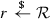denote sampling r uniformly at random from $$\mathcal {R}$$. For a binary string s, let |s| denote its length in bits. $$y\leftarrow F(x)$$ (resp.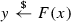) denotes y being the output of the deterministic (resp. probabilistic) function F with input x. Let $$x\leftarrow a$$ denote assigning value a to variable x. We use the wildcard $$\cdot$$ to indicate any valid input of a function.

Public Key Infrastructure For simplicity, we do not consider certificates or certificate checks but assume any public key associated with a party is supported by a public key infrastructure (PKI) and hence certified and bound to the party’s identity. That is, we omit PKI details but simply assume that the binding of public keys to party identities is publicly known by default.

### 3.1 Pseudorandom Function

For a function family $$F:\{0,1\}^\lambda \times \{0,1\}^n\rightarrow \{0,1\}^m$$, consider the following security experiment associated with an adversary $$\mathcal {A}$$. The challenger first samples a bit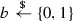. If $$b=0$$, $$\mathcal {A}$$ is given oracle access, i.e., can make queries, to $$F_k(\cdot )=F(k,\cdot )$$ where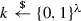. If $$b=1$$, $$\mathcal {A}$$ is given oracle access to $$f(\cdot )$$ that maps elements from $$\{0,1\}^n$$ to $$\{0,1\}^m$$ uniformly at random. In the end, $$\mathcal {A}$$ outputs a bit $$b'$$ as a guess of b. The advantage of $$\mathcal {A}$$ is defined as $$\mathbf {Adv}_F^{\mathsf {prf}}(\mathcal {A})=|\Pr [b'=1|b=0]-\Pr [b'=1|b=1]|$$, which measures $$\mathcal {A}$$’s ability to distinguish $$F_k$$ (with random k) from a random function f.

F is a pseudorandom function (PRF) if: (1) for any $$k\in \{0,1\}^\lambda$$ and any $$x\in \{0,1\}^n$$, there exists an efficient algorithm to compute F(kx); and (2) for any efficient adversary $$\mathcal {A}$$, $$\mathbf {Adv}_F^{\mathsf {prf}}(\mathcal {A})$$ is sufficiently small (e.g., roughly $$2^{-\lambda }$$).

### 3.2 Stateful Authenticated Encryption with Associated Data

We follow [13, 41] in extending the stateful authenticated encryption notion of Bellare et al.  to capture a hierarchy of stateful AEAD security notions based on different authentication levels. The following definitions are the same as , except that we exclude the length-hiding property proposed by Paterson et al.  for conciseness.

Syntax A stateful AEAD scheme $$\mathsf {sAEAD}$$ is a 4-tuple $$(\mathsf {sG},\mathsf {sI},\mathsf {sE},\mathsf {sD})$$ associated with a message space $$\mathcal {M}\subseteq \{0,1\}^{*}$$, an associated data space $$\mathcal {AD}\subseteq \{0,1\}^{*}$$, and a state space $$\mathcal {ST}\subseteq \{0,1\}^*$$. $$\mathsf {sG}$$ is a probabilistic algorithm that samples a random key k from a finite non-empty key space $$\mathcal {K}$$. $$\mathsf {sI}$$ is an algorithm that initializes the encryption and decryption states $$st_e,st_d$$. $$\mathsf {sE}$$ is a probabilistic encryption algorithm that takes as input $$k\in \mathcal {K},ad\in \mathcal {AD},m\in \mathcal {M}$$ and $$st_e$$ and outputs a ciphertext $$ct\in \{0,1\}^*$$ with an updated $$st_e$$. $$\mathsf {sD}$$ is a deterministic decryption algorithm that takes as input $$k\in \mathcal {K},ad\in \mathcal {AD},ct\in \{0,1\}^*$$ and $$st_d$$ and outputs $$m\in \mathcal {M}\cup \{\bot \}$$ with an updated $$st_d$$. The correctness requires that, for any $$k\in \mathcal {K}$$ sampled by $$\mathsf {sG}$$, any $$st_e=st_e^0,st_d=st_d^0$$ initialized by $$\mathsf {sI}$$, and any sequence of encryptions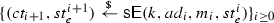, the sequence of decryptions $$\{(m_{i+1}',st_d^{i+1})\leftarrow \mathsf {sD}(k,ad,\mathsf {E}(k,ad_i,ct_i,st_d^i))\}_{i\ge 0}$$ satisfies $$m_i=m_i',i\ge 0$$.

Security Consider the following experiment with an authentication level $$al\in $$. In the beginning, run $$\mathsf {sG}$$ to generate a key k and run $$\mathsf {sI}$$ to initialize $$st_e,st_d$$. Sampleand set $$(u,v,{\mathtt {outofsync}})\leftarrow (0,0,0)$$. Then, the adversary $$\mathcal {A}$$ is given access to the following oracles:

$$\underline{\mathsf {Enc}(ad, m_0, m_1)}$$:

The above “out-of-sync” condition (see procedure 4) varies with the associated authentication level $$al\in $$. We recall the four authentication conditions $${\mathsf {cond}}_{al}$$ defined in  as follows:

$${\mathsf {cond}}_1$$::

$$(\not \exists y:(ct=sent.ct_y)\wedge (ad=sent.ad_y))$$

$${\mathsf {cond}}_2$$::

$$(\not \exists y:(ct=sent.ct_y)\wedge (ad=sent.ad_y))\vee (\exists w<v:ct=rcvd.ct_w)$$

$${\mathsf {cond}}_3$$::

$$(\not \exists y:(ct=sent.ct_y)\wedge (ad=sent.ad_y))\vee (\exists w,x,y:(w<v)\wedge (sent.ct_x=rcvd.ct_w)\wedge (sent.ct_y=ct)\wedge (x\ge y))$$

$${\mathsf {cond}}_4$$::

$$(u<v)\vee (ct\ne sent.ct_v)\vee (ad\ne sent.ad_v)$$

Note that $${\mathsf {cond}}_{al}$$ constrains the adversary’s ability to make an “out-of-sync” $$\mathsf {Dec}$$ query (which is necessary to get a non-$$\bot$$ value). That is, the more easily $${\mathsf {cond}}_{al}$$ can be satisfied, the more powerful the adversary is, and hence the higher authentication level the security experiment captures. In particular, $${\mathsf {cond}}_1$$ corresponds to the lowest authentication level that only guarantees no forgeries; $${\mathsf {cond}}_2$$ adds a clause to additionally capture replays; $${\mathsf {cond}}_3$$ extends $${\mathsf {cond}}_2$$ to further capture reordering (note that $${\mathsf {cond}}_3$$ constrained on $$x=y$$ is equal to $${\mathsf {cond}}_2$$); finally, $${\mathsf {cond}}_4$$ corresponds to the highest authentication level that prevents forgeries, replays, reordering, and dropping.

In the end, $$\mathcal {A}$$ outputs a bit $$b'$$. The stateful AEAD scheme $$\mathsf {sAEAD}$$ is secure with authentication level al if and only if $$\mathbf {Adv}_\mathsf {sAEAD}^{\mathsf {aead\text {-}}{al}}(\mathcal {A})=|2\Pr [b=b']-1|$$ is sufficiently small (e.g., roughly $$2^{-\log {|\mathcal {K}|}}$$) for any efficient adversary $$\mathcal {A}$$.

## 4 Multi-stage Authenticated and Confidential Channel Establishment

In this section, we define the syntax and two security models for multi-stage authenticated and confidential channel establishment (msACCE) protocols.

### 4.1 Protocol Syntax

Our msACCE protocol is an extension to the quick connection (QC) protocol proposed by Lychev et al.  and the multi-stage key exchange (MSKE) protocol proposed by Fischlin and Günther  (and further developed by [24, 25, 27, 47]). Even though the authors of  claimed their QC protocol syntax to be general, TLS 1.3 does not fit it well because TLS 1.3 has two initial keys and one final key in 0-RTT resumption while QC captures only one initial key. On the other hand, the MSKE protocol and its extensions focus only on the key exchange phases.

Our msACCE protocol syntax inherits many parts of the QC protocol syntax but extends it to a multi-stage structure and additionally covers session resumptions (formally, unlike QC), session resets, and header-only packets exchanged in secure channel phases. msACCE also employs a more general stateful authenticated encryption syntax (rather than a nonce-based one) to capture the encryption schemes of both TLS 1.3 and QUIC.

The detailed protocol syntax is defined below.

A msACCE protocol is an interactive protocol between a client and a server. The protocol consists of one or more stages and each stage consists of two phases (that are formally defined later): key exchange and secure channel. The client and server establish keys in key exchange phases and exchange messages encrypted and decrypted with these keys in secure channel phases. Messages are exchanged via packets. A packet consists of source and destination IP addresses $$\text {IP}_{\mathsf {s}},\text {IP}_{\mathsf {d}}\in \{0,1\}^{32}\cup \{0,1\}^{128}$$, a header, and a payload. Each party P has a unique IP address $$\text {IP}_P$$. Note that for the network layer, we only consider the Internet Protocol and its IP address header fields because our model mainly focuses on the application and transport layers and additionally only captures the IP-spoofing attack. Both parties can keep static state (shared among several sessions) and volatile state (used only within one session). All states are initialized to the empty string $$\varepsilon$$.

The protocol is associated with the security parameter $$\lambda \in {{\mathbb N}}_+$$, a key generation algorithm $${\mathsf {Kg}}$$ that takes as input $$1^\lambda$$ and outputs a public and secret key pair, a header space (for transport and application layers) $$\mathcal {H}\subseteq \{0,1\}^{*}$$ that may exclude some header fields of the analyzed protocol (e.g., port numbers, checksums, etc.) if they do not affect the security analysis, a payload space $$\mathcal {PD}\subseteq \{0,1\}^*$$, header and payload spaces for reset packets (described later) $$\mathcal {H}_{\mathsf {rst}}\subseteq \mathcal {H},\mathcal {PD}_{\mathsf {rst}}\subseteq \mathcal {PD}$$, a resumption state space $$\mathcal {RS}\subseteq \{0,1\}^*$$, and a stateful AEAD scheme $$\mathsf {sAEAD}=(\mathsf {sG},\mathsf {sI},\mathsf {sE},\mathsf {sD})$$ (with a message space $$\mathcal {M}\subseteq \{0,1\}^{*}$$, an associated data space $$\mathcal {AD}\subseteq \{0,1\}^{*}$$, and a state space $$\mathcal {ST}\subseteq \{0,1\}^*$$). The protocol is also associated with three disjoint message spaces $$\mathcal {M}_{\mathsf {KE}},\mathcal {M}_{\mathsf {SC}},\mathcal {M}_{\mathsf {pRST}}\subseteq \mathcal {M}$$, where $$\mathcal {M}_{\mathsf {KE}},\mathcal {M}_{\mathsf {SC}}$$ consist of messages encrypted in key exchange and secure channel phases, respectively, and $$\mathcal {M}_{\mathsf {pRST}}$$ consists of pre-reset messages (described later) encrypted in a secure channel phase. Note that disjointness of these message spaces is a reasonable assumption as practical protocols (such as those in Table 1) enforce different leading bits for different types of messages.

During the protocol’s execution, the server keeps a (static) local time state $${\mathtt {loct}}$$ that represents its current time period, which is initialized to 0 and gets incremented (by one) periodically. The server may keep a (static) configuration state $${\mathtt {scfg}}$$ that is updated whenever $${\mathtt {loct}}$$ is incremented (e.g., for security reasons); if so, the protocol is further associated with a server configuration generation function $$\mathsf {scfg\_gen}$$, which takes as input $$1^\lambda$$, the server secret key (generated by $${\mathsf {Kg}}$$), and a timestamp (the content of $${\mathtt {loct}}$$), then outputs a new server configuration state. Note that in the QC protocol syntax  $${\mathtt {scfg}}$$ is also updated at the beginning of each time period, but there the notion of time was modeled rather informally. We refer to [5, 64] for “timed” models proposed to analyze authentication and key exchange protocols whose security relies crucially on time synchronization, but this is not the case for the protocols analyzed in this work (for which timestamps, if any, are essentially only used by servers to expire resumption tokens). In order to not further complicate our already complex model, we choose to simplify our time modeling by replacing timestamps with local $${\mathtt {loct}}$$ counters. The advancement of (server-side) time periods is triggered by the $${\mathsf {NextTP}}$$ oracle as described later in our security model.

A reset packet enables the sender, who lost its volatile session state due to some error condition (e.g., server reboots, denial-of-service attacks, etc.) and hence lost its previous connection with the receiver, to reset (i.e., terminate without completing) their session. A pre-reset message (e.g., a reset token in UDP+QUIC[TLS]) may have been sent to the receiver in a secure channel phase as a pre-reset packet before the sender lost its state, in order to let the receiver verify the sender’s reset packet, as shown in Fig. 5. Note that a pre-reset message can also be carried within an encrypted key exchange packet; we consider only the case where it is encrypted as a separate secure channel packet to get clean security models described later. For simplicity, we assume each party generates at most one pre-reset message for each session. A non-reset packet is a packet that is not a reset packet.

We say a party rejects a packet if its processing the packet leads to an error (defined according to the protocol), and accepts it otherwise.

At the beginning of the protocol’s execution, each party takes as input a list of messages $$\mathcal {M}^{\mathsf {snd}}=(M_1,\ldots ,M_l),M_i\in \mathcal {M}_{\mathsf {SC}},l\in {{\mathbb N}}$$ (where the total message length $$|\mathcal {M}^{\mathsf {snd}}|$$ is polynomial in $$\lambda$$) as well as the other party’s IP address. Note that in reality the exchanged messages may depend on each other, but modeling that complicates our already complex protocol syntax and it has no real implications on our security analysis since as we will show the adversary chooses all secure channel messages. Furthermore, for simplicity we consider transmission of atomic messages rather than a data stream, where the latter was modeled in  and later extended to capture multiplexing .

The protocol has two modes: full and resumption. Its corresponding executions are referred to as the full and resumption sessions. Each resumption session is associated with a unique previous full session, and we say the resumption session resumes its associated full session. At the beginning of a full session, the server takes as input its associated public and secret key pair (generated by $${\mathsf {Kg}}$$) and the client takes the server’s public key as input. (This implies that the client knows the identifier of its intended communicating peer when the protocol starts, so our protocol is analyzed in the pre-specified peer model .) For a resumption session, each party additionally takes as input its own (static) resumption state $$rs\in \mathcal {RS}$$ (set in the associated full session). In either case, the client sends the first packet to start a session and each party may set its static state if it is not set yet. In particular, if $$\mathsf {scfg\_gen}$$ is defined and the server’s configuration state $${\mathtt {scfg}}$$ has not been set, the server sets $${\mathtt {loct}}$$ to 0 and runs $$\mathsf {scfg\_gen}$$ to set its $${\mathtt {scfg}}$$, which may be further updated during the protocol’s execution.

A D-stage (for an arbitrary $$D\in {{\mathbb N}}_+$$) msACCE protocol consists of D successive stages and each d-th ($$d\in [D]$$) stage consists of one or two phases described as follows:

(1) Key Exchange At the end of this phase, each party sets its d-th stage key $$k^d = (k^d_c, k^d_s)$$. At most one of $$k^d_c$$ and $$k^d_s$$ could be $$\bot$$ (i.e., unused); this captures the case where a 0-RTT key consists of only a client encryption key while the server encryption key does not exist. If this phase belongs to the final stage of a full session, each party can send additional key exchange messages encrypted with $$k^d_c$$ or $$k^d_s$$ after setting $$k^d$$, e.g., post-handshake key exchange messages that are used for session resumption, post-handshake authentication, key updates, etc.; at the end of this phase, each party sets its own resumption state.

(2) Secure Channel This phase is mandatory for the final stage but optional for other stages. In this phase, the parties can exchange messages from their input lists as well as pre-reset messages, encrypted and decrypted using the associated stateful $${\text {AEAD}}$$ scheme with $$k^d$$. (For simplicity, we do not consider key updates inside the secure channel, which was modeled in .) The client uses $$k^d_c$$ to encrypt and the server uses it to decrypt, whereas the server uses $$k^d_s$$ to encrypt and the client uses it to decrypt. They may also send reset or header-only packets. At the end of this phase, each party outputs a list of received messages (which may be empty).

Each message exchanged between the parties must belong to some unique phase at some unique stage. One stage’s second phase and the next stage’s first phase may overlap, and the two phases in the final stage may also overlap. We call the final stage key the session key and the other stage keys the interim keys.

Correctness Consider a client and a server running a D-stage msACCE protocol in either mode without sending any reset packet. Each party’s input message list $$\mathcal {M}^{\mathsf {snd}}$$, in which the messages are sent among D stages according to any possible partitioning $$\mathcal {M}^{\mathsf {snd}}=\mathcal {M}^{\mathsf {snd}}_1,$$ $$\ldots ,\mathcal {M}^{\mathsf {snd}}_D$$, is equal to the other party’s total output message list $$\mathcal {M}^{\mathsf {rcv}}=\mathcal {M}^{\mathsf {rcv}}_1,\ldots ,\mathcal {M}^{\mathsf {rcv}}_D$$, in which the message order is preserved. Each party terminates its session upon receiving the other party’s reset packet.

Relations to Prior ACCE-type Protocols With our more general protocol syntax, the ACCE  and QC  protocols can be classified into 1-stage and 2-stage msACCE protocols, respectively.

### 4.2 Security Models

We propose two security models, respectively, for basic authenticated and confidential and novel packet authentication. Our models do not consider the key exchange and secure channel phases independently, as was the case in the MSKE model (and its extensions) used by some previous QUIC and TLS 1.3 security analyses [14, 24,25,26,27, 29, 47], because QUIC’s key exchange and secure channel phases are inherently inseparable and the TLS 1.3 full handshake does not fit into a composability framework, as discussed in [25, 49].

(1) msACCE Standard Security Model

In this msACCE standard (msACCE-std) security model, we consider the standard security goals for msACCE protocols: Server Authentication and Channel Security. Note that for simplicity our msACCE-std model focuses on the most common unilateral server authentication, but it can be extended to mutual authentication, e.g., as described in .

Our msACCE-std model is based on the standard security portion of the QACCE model , but extends it to capture four authentication levels (following those of stateful authenticated encryption schemes) and any number of stages. Recall that QACCE considers only two stages within a session and hence cannot be used to analyze the TLS 1.3 0-RTT resumption. Inspired by the “timed” models proposed by [5, 64], our model introduces a new $${\mathsf {NextTP}}$$ oracle (defined later) to formally capture the advancement of time periods on the server side, which was not modeled properly in QACCE. Finally, like QACCE and other previous models, we consider a very powerful adversary who can control communications between honest parties, can adaptively learn their stage keys, and can adaptively corrupt servers to learn their long-term keys and secret states.

The detailed security model is defined below.

Protocol Entities The set of parties $$\mathcal {P}$$ consists of two disjoint type of parties: clients $$\mathcal {C}$$ and servers $$\mathcal {S}$$, i.e., $$|\mathcal {P}|=|\mathcal {C}|+|\mathcal {S}|$$.

Session Oracles To capture multiple sequential and parallel protocol executions, each party $$P\in \mathcal {P}$$ is associated with a set of session oracles $$\pi _{P}^{1},\pi _P^2,\ldots$$, where $$\pi _P^i$$ models P executing a protocol instance in session $$i\in {{\mathbb N}}_+$$.

Session Identifiers As part of the security model, session identifiers are used to model entity authentication, session key confirmation, and handshake integrity. Compared to the general definition of matching conversations [9, 37] used for modeling entity authentication, a session identifier $${\mathtt {sid}}$$ is defined according to the protocol specifications and security goals, often as a subset of the entire communication transcript. For instance, QUIC’s $${\mathtt {sid}}$$ in QACCE  is defined as the second-round key exchange messages, i.e., ClientHello and ServerHello, while the first-round messages are excluded to allow for valid but different source-address tokens or signatures. Similarly, TLS 1.2’s $${\mathtt {sid}}$$ in ACCE  is defined as the first three key exchange messages, while the rest are excluded to allow for valid but different encrypted Finished messages. A msACCE protocol may have different session identifiers in full and resumption modes, but for simplicity we use the same notation $${\mathtt {sid}}$$.

Peers and Partners We say a client oracle and a server oracle are each other’s peer if they share the same session identifier $${\mathtt {sid}}$$; we say they are each other’s partner if they share the same first-stage session identifier $${\mathtt {sid}}_1$$ (i.e., $${\mathtt {sid}}$$ restricted to the first stage), which intuitively means that they set the first stage key with each other. Note that a client oracle may have more than one partner if $${\mathtt {sid}}_1$$ consists of only message(s) sent from the client oracle, which can be replayed to the same server to establish multiple (identical) first-stage keys. (In practice, such 0-RTT replay attacks can be mounted to different servers using the same public–secret key pair; however, 0-RTT key exchange message(s) replayed to other servers using different public–secret key pairs should be rejected.) Therefore, a session oracle’s partner may not be its final unique communication peer.

Security Experiment In order to define security, we consider the following security experiment run between a challenger and an adversary $$\mathcal {A}$$. At the beginning of the experiment, the challenger initializes the states of all parties and then runs $${\mathsf {Kg}}$$ for all servers to generate their public–secret key pairs. The static states of all parties are properly set and in particular $$\mathsf {scfg\_gen}$$ (if defined) is executed for each server (where $${\mathtt {loct}}\leftarrow 0$$) to set its $${\mathtt {scfg}}$$ state. All other states are initialized to the empty string $$\varepsilon$$. The experiment is associated with an authentication level $$al\in $$ for channel security. Each oracle $$\pi _P^i$$ is associated with a random bit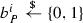. Let $$N\in {{\mathbb N}}_+$$ denote the maximum number of msACCE protocol instances for each party and $$D\in {{\mathbb N}}_+$$ denote the maximum number of stages in each session. The adversary $$\mathcal {A}$$ is given all public keys and IP addresses associated with all parties and then interacts with session oracles and parties via the following queries:

• $${\mathsf {Connect}}(\pi _C^i,S)$$, for $$C\in \mathcal {C},S\in \mathcal {S},i\in [N]$$. This query allows the adversary to ask a specified client oracle to start a full session with a specified server. $$\pi _C^i$$ outputs the first packet it would send to S in a full session according to the protocol. This output packet is returned to $$\mathcal {A}$$ instead of S. After this query, we say S is the intended server of $$\pi _C^i$$. The session oracle created in this query (i.e., $$\pi _C^i$$) must have never been used before.

• $${\mathsf {Resume}}(\pi _C^i,S,i')$$, for $$C\in \mathcal {C},S\in \mathcal {S}$$, $$i,i'\in [N]$$, $$i'<i$$. This query allows the adversary to ask a specified client oracle to start a resumption session with a specified server to resume a specified full session between the two parties, if the associated previous client oracle has set its resumption state. $$\pi _C^i$$ inputs the resumption state set by $$\pi _C^{i'}$$ and outputs the first packet it would send to S in a resumption session according to the protocol, where the output packet is returned. This query is only allowed if $$\pi _C^{i'}$$ has set its resumption state. The session oracle created in this query (i.e., $$\pi _C^i$$) must have never been used before.

• $${\mathsf {Send}}(\pi _P^i,pkt)$$, for $$P\in \mathcal {P},i\in [N],pkt\in \{0,1\}^*$$. This query allows the adversary to send any packet to a specified session oracle and get its response in a key exchange phase. If $$\pi _P^i$$ is in a key exchange phase, pkt is sent to $$\pi _P^i$$ and the response is returned, otherwise, returns $$\bot$$.

• $${\mathsf {Reveal}}(\pi _P^i, d)$$, for $$P\in \mathcal {P},i\in [N],d\in [D]$$. This query allows the adversary to learn any stage key of a specified session oracle. The contents of $$\pi _P^i$$’s d-th stage key state $$k^d$$ is returned. After this query, we say $$k^d$$ was revealed.

• $${\mathsf {Corrupt}}(S)$$, for $$S\in \mathcal {S}$$. This query allows the adversary to learn the long-term secret and static state of a specified server. The long-term secret key, configuration state (if any), resumption states, and other static state of S are returned. After this query, we say S was corrupted.

• $${\mathsf {NextTP}}(S)$$, for $$S\in \mathcal {S}$$. This query allows the adversary to advance the time period of a specified server. If $${\mathtt {scfg}}$$ is defined, it further allows the adversary to reconfigure the specified server. The local time state $${\mathtt {loct}}$$ of S is incremented by one and $$\mathsf {scfg\_gen}$$ (if defined) is executed to update the configuration state $${\mathtt {scfg}}$$; the value of $${\mathtt {loct}}$$ is returned. If $$\mathsf {scfg\_gen}$$ is executed, we say S was reconfigured (at time period $${\mathtt {loct}}$$).

• $${\mathsf {Encrypt}}(\pi _P^i, d, ad, m_0, m_1)$$, for $$P\in \mathcal {P},i\in [N],d\in [D],ad\in \mathcal {AD},m_0,m_1\in \mathcal {M}_{\mathsf {SC}}\cup \mathcal {M}_{\mathsf {pRST}}\cup \{{\mathtt {rst}}\}$$. This query allows the adversary to specify any associated data and any two secure channel or pre-reset messages of the same length, and then get the ciphertext of one message determined by $$b_P^{i}$$, the random bit associated with a specified session oracle at a specified stage. This query also keeps a state(initialized to $$\varepsilon$$) for $$\pi _P^i$$ to store one of the specified pre-reset messages determined by $$b_P^{i}$$ and allows the adversary to retrieve it on request. (Recall that each oracle has at most one pre-reset message, so this query stores only the first pre-reset message and rejects others.) The detailed procedures are listed as follows:

• $${\mathsf {Decrypt}}(\pi _P^i,d,ad,ct)$$, for $$P\in \mathcal {P},i\in [N],d\in [D],ad\in \mathcal {AD},ct\in \{0,1\}^*$$. This query allows the adversary to specify any associated data and any ciphertext to be decrypted by the partner(s) of a specified session oracle at a specified stage, and then get the secret bit $$b_P^{i}$$ if the decrypted message is a valid secure channel or pre-reset message and this query is “out-of-sync” (defined below); otherwise, it gets $$\bot$$. The detailed procedures are listed as follows:

Advantage Measures An adversary $$\mathcal {A}$$ against a msACCE protocol $$\Pi$$ in msACCE-std has the following advantage measures (that each takes the security parameter as an implicit input).

Server Authentication. We define $$\mathbf {Adv}_\Pi ^{\mathsf {s\text {-}auth}}(\mathcal {A})$$ as the probability that there exist a client oracle $$\pi _C^i$$ and its intended server S such that the following conditions hold:

1. 1.

$$\pi _C^i$$ has set its session key;

2. 2.

S was not corrupted before $$\pi _C^i$$ set its session key;

3. 3.

No interim keys of $$\pi _C^i$$ or its partner(s) were revealed;

4. 4.

There is no unique server oracle $$\pi _S^j$$ that is $$\pi _C^i$$’s peer.

The above captures the attacks in which the adversary impersonates a server to make the client mistakenly believe that it shares the session key with the server. Note that the above condition 3 can be relaxed to allow revealing all but the first stage key of a $$\pi _C^i$$’s partner that observes the same session identifier at only the first stage but not the next one, because such a partner’s key exchange message is never received by $$\pi _C^i$$. Such condition relaxation also holds for other security notions defined in this work. We also note that Server Authentication does not depend on the authentication level al. To see this, consider a weaker Server Authentication notion defined in the same way except its security experiment has no $${\mathsf {Decrypt}}$$ query and the $${\mathsf {Encrypt}}$$ query takes only one message as input. One can easily reduce our Server Authentication security with an arbitrary authentication level al to the above weaker Server Authentication security. Therefore, Server Authentication is al-independent.

(level-al)Channel Security. We define $$\mathbf {Adv}_\Pi ^{\mathsf {cs\text {-}}{al}}(\mathcal {A})$$ as $$|2\Pr [b_P^{i}=b']-1|$$, where $$al\in $$ is the specified authentication level and $$(P,i,b')$$ is output by $$\mathcal {A}$$, such that the following conditions hold:

1. 1.

If $$P=S\in \mathcal {S}$$, $$\pi _S^i$$ has a peer $$\pi _C^j$$; if $$P=C\in \mathcal {C}$$, denote S as $$\pi _C^i$$’s intended server;

2. 2.

If S was corrupted, then 1) S was corrupted after $$\pi _P^i$$ set its last stage key and 2) for any (d-th) stage key of $$\pi _P^i$$ that is not required to provide forward secrecy, no $${\mathsf {Encrypt}}(\pi _P^i,d,\cdot ,\cdot ,\cdot )$$ queries were made before S was (if ever) reconfigured after $$\pi _P^i$$ set its d-th stage key.

3. 3.

No stage keys of $$\pi _P^i$$ or its partner(s) were revealed;

4. 4.

If an $${\mathsf {Encrypt}}(\pi _P^i,\cdot ,\cdot ,\cdot ,\cdot )$$ query was made on two distinct pre-reset messages, then later no $${\mathsf {Encrypt}}(\pi _P^i,\cdot ,\cdot ,{\mathtt {rst}},{\mathtt {rst}})$$ queries were made.

The above captures the attacks in which the adversary compromises the confidentiality or integrity of secure channel messages without revealing stage keys or the hidden pre-reset message or corrupting the server before the client sets its last stage key (which may not be the session key). If some stage key is not supposed to provide forward secrecy, the adversary is further prevented from accessing the communication of that stage if the server was not reconfigured before it was corrupted.

(2) msACCE Packet-Authentication Security Model

In this msACCE packet-authentication (msACCE-pauth) security model, we consider security goals related to packet authentication beyond those captured by the msACCE-std model. Note that msACCE-std essentially focuses only on the packet fields in the application layer, while msACCE-pauth further covers transport-layer headers and IP addresses.

First, we consider IP spoofing prevention as with the QACCE model, but, as illustrated later, generalize one of the QACCE queries to additionally capture IP spoofing attacks in the full sessions. Then, more importantly, we define four novel packet-level security notions (elaborated later): KE Header Integrity, KE Payload Integrity, SC Header Integrity, and Reset Authentication, which enable a comprehensive and fine-grained security analysis of layered protocols.

In particular, KE Header and Payload Integrity, respectively, capture the header and payload integrity of key exchange packets. Such security issues have not been investigated before and, as we show later, lead to new availability attacks for both TFO+TLS 1.3 and UDP+QUIC. Furthermore, we employ SC Header Integrity to capture the header integrity of non-reset packets in secure channel phases. Note that, unlike the availability attacks shown in , successful attacks breaking our security notions are harder or impossible to detect by the client as they do not affect the client’s session key establishment, so these attacks could be more harmful in this sense. Finally, our model captures malicious undetectable session resets in a secure channel phase with Reset Authentication.

As with the msACCE-std model, msACCE-pauth captures multiple stages and considers a very powerful adversary. It also inherits the same definitions of protocol entities, session oracles, session identifiers, peers, and partners. The security experiment and advantage measures are defined below.

Security Experiment Consider the same experiment setups as in msACCE-std, except that no random bit $$b_P^{i}$$ is needed. The adversary $$\mathcal {A}$$ is given all the public parameters and interacts with the session oracles via the same $${\mathsf {Connect}},{\mathsf {Resume}},{\mathsf {Send}},{\mathsf {Reveal}},{\mathsf {Corrupt}},{\mathsf {NextTP}}$$ queries as in the msACCE-std model, where $${\mathsf {Encrypt}}$$ and $${\mathsf {Decrypt}}$$ queries are not needed because msACCE-pauth does not consider data confidentiality and integrity that are already captured by msACCE-std, as well as the following:

• $${\mathsf {Connprivate}}(\pi _C^i,\pi _S^j,{\mathtt {cmp}})$$, for $$C\in \mathcal {C}$$, $$S\in \mathcal {S}$$, $$i,j\in [N],{\mathtt {cmp}}\in \{0,1\}$$. This query allows the adversary to run a complete or partial full session between any specified client and server oracles without observing their communication. This query always returns $$\bot$$. If $${\mathtt {cmp}}=1$$, $$\pi _C^i$$ and $$\pi _S^j$$ run a complete full session privately without showing their communication to $$\mathcal {A}$$. If $${\mathtt {cmp}}=0$$, $$\pi _C^i$$ and $$\pi _S^j$$ run a partial full session privately such that the last packet sent from $$\pi _C^i$$ right before $$\pi _S^j$$ sets its first stage key is blocked. Note that this query extends the QACCE $${\mathsf {Connprivate}}$$ query  to model IP-spoofing attacks targeting both full and resumption sessions, with help of an input flag $${\mathtt {cmp}}$$.

• $${\mathsf {Pack}}(\pi _P^i, ad, m)$$, for $$P\in \mathcal {P},i\in [N],ad\in \mathcal {AD},m\in \mathcal {M}_{\mathsf {SC}}\cup \mathcal {M}_{\mathsf {pRST}}\cup \{{\mathtt {prst}},{\mathtt {rst}}\}$$. This query allows the adversary to specify any associated data and any message in a secure channel phase, then get the packet output by a specified session oracle. It also allows the adversary to ask a specified session oracle to output its pre-reset packet, which is either for a specified pre-reset message (if $$m\in \mathcal {M}_{\mathsf {pRST}}$$) or for a real one (hidden from the adversary) generated according to the protocol (if $$m={\mathtt {prst}}$$), or output its reset packet (if $$m={\mathtt {rst}}$$). The detailed procedures are listed as follows:

• $${\mathsf {Deliver}}(\pi _P^i,pkt)$$, for $$P\in \mathcal {P},i\in [N],pkt\in \{0,1\}^*$$. This query allows the adversary to deliver any packet to a specified session oracle and get its response in a secure channel phase.

If $$\pi _P^i$$ is in a secure channel phase, pkt is delivered to $$\pi _P^i$$ and its response (if any) is returned, otherwise, returns $$\bot$$.

Advantage Measures An adversary $$\mathcal {A}$$ against a msACCE protocol $$\Pi$$ in msACCE-pauth has the following associated advantage measures (that each takes the security parameter as an implicit input).

IP-Spoofing Prevention. We define $$\mathbf {Adv}_\Pi ^{\mathsf {ipsp}}(\mathcal {A})$$ as the probability that there exist a client oracle $$\pi _C^i$$ and a server oracle $$\pi _S^j$$ such that the following conditions hold:

1. 1.

$$\pi _S^j$$ has set its first stage key right after a $${\mathsf {Send}}(\pi _S^j,(\text {IP}_C,\text {IP}_S,\cdot ,\cdot ))$$ query;

2. 2.

S was not corrupted before $$\pi _S^j$$ set its first stage key;

3. 3.

For any session oracle $$\pi _S^y$$ of S that shares the same time period (i.e., $${\mathtt {loct}}$$ value) with $$\pi _S^j$$, the only queries made by $$\mathcal {A}$$ about C (before $$\pi _S^j$$ set its first stage key) were:

• $${\mathsf {Connprivate}}(\pi _C^x,\pi _S^y,\cdot )$$ for some $$x\in [N]$$, or

• $${\mathsf {Send}}(\pi _S^y,(\text {IP}_C,\text {IP}_S,\cdot ,\cdot ))$$, where $$(\text {IP}_C,\text {IP}_S,\cdot ,\cdot )$$ was the last packet received by $$\pi _S^y$$ right before it set its first stage key.

The above captures the attacks in which the adversary fools a server into accepting a spurious connection request seemingly from an impersonated client, without observing any previous communication between the client and server in the same time period associated with the accepting server. Such attacks can lead to exhaustion of server resources, i.e., denial of service, by triggering excessive stage key derivation and probably 0-RTT data processing.

KE Header Integrity. We define $$\mathbf {Adv}_\Pi ^{\mathsf {int\text {-}keh}}(\mathcal {A})$$ as the probability that there exist a client oracle $$\pi _C^i$$ and a server oracle $$\pi _S^j$$ such that the following conditions hold:

1. 1.

$$\pi _C^i$$ has set its session key and has a peer $$\pi _S^j$$;

2. 2.

S was not corrupted before $$\pi _C^i$$ set its session key;

3. 3.

No interim keys of $$\pi _C^i$$ or its partner(s) were revealed;

4. 4.

In a key exchange phase before $$\pi _C^i$$ set its session key, $$\pi _C^i$$ (resp. $$\pi _S^j$$) accepted a packet with a header that was not output by $$\pi _S^j$$ (resp. $$\pi _C^i$$).

The above captures the attacks in which the adversary modifies the protocol header of a key exchange packet of the communicating parties without affecting the client setting its session key. In the above definition, we assume that a client sets its session key immediately after sending its last key exchange packet(s) (if any). Then, a forged packet that leads to a successful attack cannot be any of these last packet(s), which have not yet been sent to the server. The same assumption is made for KE Payload Integrity defined below.

KE Payload Integrity. We define $$\mathbf {Adv}_\Pi ^{\mathsf {int\text {-}kep}}(\mathcal {A})$$ as the probability that there exist a client oracle $$\pi _C^i$$ and a server oracle $$\pi _S^j$$ such that the same (1)$$\sim$$(3) conditions as in the above KE Header Integrity notion hold and the following holds:

1. 4.

In a key exchange phase before $$\pi _C^i$$ set its session key, $$\pi _C^i$$ (resp. $$\pi _S^j$$) accepted a packet with a payload that was not output by $$\pi _S^j$$ (resp. $$\pi _C^i$$).

The above captures the attacks in which the adversary modifies the payload of a key exchange packet of the communicating parties without affecting the client setting its session key. Note that the above notion can be trivially achieved if the session identifier includes the entire key exchange transcript (excluding some headers), which is the case for TLS 1.3. However, some other protocols like QUIC only have partial transcripts as their session identifiers.

SC Header Integrity. We define $$\mathbf {Adv}_\Pi ^{\mathsf {int\text {-}h}}(\mathcal {A})$$ as the probability that $$\mathcal {A}$$ outputs (Pi) such that the same (1)$$\sim$$(3) conditions as in the Channel Security notion hold and the following holds:

1. 4.

In a secure channel phase, a partner of $$\pi _P^i$$ accepted a non-reset packet with a header that was not output by $$\pi _P^i$$ (via $${\mathsf {Pack}}$$ queries), or a partner of $$\pi _P^i$$ accepted a non-reset header-only packet.

The above captures the attacks in which the adversary creates a valid non-reset secure channel packet by forging the protocol header without breaking any Channel Security conditions. Note that in the above security notion an invalid header forgery is detected immediately after the malicious packet is received and processed, while the detection of invalid packet forgeries in a key exchange phase (e.g., for plaintext packets) can be delayed to the point when the client sets its session key, according to the definitions of KE Header and Payload Integrity.

We define $$\mathbf {Adv}_\Pi ^{\mathsf {rst\text {-}auth}}(\mathcal {A})$$ as the probability that $$\mathcal {A}$$ outputs (Pi) such that the same (1)$$\sim$$(3) conditions as in the Channel Security notion hold and the following holds:

1. 4.

In a secure channel phase, a partner of $$\pi _P^i$$ accepted a reset packet and 1) if $$P\in \mathcal {S}$$ then P was not corrupted before $$\pi _P^i$$ accepted that reset packet and 2) the $${\mathsf {Pack}}(\pi _P^i,\cdot ,m)$$ queries made by $$\mathcal {A}$$ were only for $$m\in \mathcal {M}_{\mathsf {SC}}\cup \{{\mathtt {prst}}\}$$ (i.e., $$m\not \in \mathcal {M}_{\mathsf {pRST}}\cup \{{\mathtt {rst}}\}$$).

The above captures the attacks in which the adversary forges a valid reset packet without breaking any Channel Security conditions or corrupting the secret static state used to generate the reset packet. Note that such attacks are undetectable by the accepting party that resets the session, as opposed to a network attacker that simply drops packets which may eventually lead to a session reset.

Remark about msACCE security model completeness and low-layer integrity Note that the payload integrity in secure channels is captured by Channel Security. Our msACCE-std and msACCE-pauth models completely capture the authentication (or integrity) of all packet fields in the transport and application layers. Furthermore, msACCE-pauth captures (network-layer) IP-Spoofing Prevention against weaker off-path attackers (i.e., those can only inject packets without observing the communication), but leaves other integrity attacks on low layers (e.g., network, link, and physical layers) uncovered. Such attacks may affect packet forwarding, node-to-node data transfer, or raw data transmission, which are outside the scope of our work.

## 5 Provable Security Analysis

Equipped with msACCE security models, we now analyze and compare the security of TFO+TLS 1.3, UDP+QUIC, and UDP+QUIC[TLS]. The security results are summarized in Table 2 (recalled as Table 3). As mentioned in Introduction, by [27, 49] results, none of the above protocols achieves forward secrecy for 0-RTT keys or protects against 0-RTT data replays (which contribute to the first two rows in the table). We now move to the detailed analyses and start with TFO+TLS 1.3.

### 5.1 TLS 1.3 over TFO

(1) Protocol Description

Referring to msACCE protocol syntax, the TFO+TLS 1.3 2-RTT full handshake (see Fig. 2a) is a 2-stage msACCE protocol in the full mode and the TFO+TLS 1.3 0-RTT resumption handshake (see Fig. 2b) is a 3-stage msACCE protocol in the resumption mode. Note that we focus only on the main components of the handshakes and omit more advanced features such as 0.5-RTT data, client authentication, and post-handshake messages (except NewSessionTicket). In a full handshake, the initial keys are set after sending or receiving ServerHello and the final keys (i.e., session keys) are set after sending or receiving ClientFinished (but only handshake messages up to ServerFinished are used for final key generation). In a 0-RTT resumption handshake, the parties set 0-RTT keys to encrypt or decrypt 0-RTT data, after sending or receiving ClientHello.

According to the TFO and TLS 1.3 specifications [17, 60], the TFO+TLS 1.3 header contains the TCP header (see Fig. 1). We ignore some uninteresting header fields such as port numbers and the checksum because modifying them only leads to redirected or dropped packets. Such adversarial capabilities are already considered in the msACCE security models. We thus define the header space $$\mathcal {H}$$ as containing the following TCP header fields: a 32-bit sequence number $${\mathtt {sqn}}$$, a 32-bit acknowledgment number $${\mathtt {ack}}$$, a 4-bit data offset $${\mathtt {off}}$$, a 6-bit reserved field $${\mathtt {resvd}}$$, a 6-bit control bits field $${\mathtt {ctrl}}$$, a 16-bit window $${\mathtt {window}}$$, a 16-bit urgent pointer $${\mathtt {urgp}}$$, a variable-length ($$\le$$ 320-bit) padded options $${\mathtt {opt}}$$. For encrypted packets, $$\mathcal {H}$$ additionally contains TLS 1.3 record header fields: an 8-bit type $${\mathtt {type}}$$, a 16-bit version $${\mathtt {ver}}$$, and a 16-bit length $${\mathtt {len}}$$. We further define reset packets as those with the RST bit (i.e., the 4-th bit of $${\mathtt {ctrl}}$$) set to 1.

In “Appendix A,” we formalize TLS 1.3’s stateful AEAD scheme $$\mathsf {sAEAD}_\text {TLS}$$ based on its underlying nonce-based AEAD scheme (instantiated with AES-GCM or other schemes as documented in ). The associated data space $$\mathcal {AD}$$ contains the TLS 1.3 record header. TLS 1.3 enforces different content types for encrypted key exchange and secure channel messages. For simplicity, we define $$\mathcal {M}_{\mathsf {KE}}$$ and $$\mathcal {M}_{\mathsf {SC}}$$ as consisting of bit strings differing in their first bits. Note that $$\mathcal {M}_{\mathsf {pRST}}=\varnothing$$.

For TFO+TLS 1.3, $$\mathsf {scfg\_gen}$$ erases the server’s resumption states (if any) and refreshes the (static) key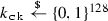used by the TFO cookie generation function F. If the TLS 1.3 session ticket is implemented as a self-encrypted and self-authenticated value, $$\mathsf {scfg\_gen}$$ also refreshes the server’s (static) session ticket encryption key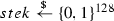used by an authenticated encryption scheme (e.g., AES-GCM ).

We refer to Fig. 6 for the remaining details of TFO, where F is instantiated with an AES-128 block cipher with output truncated to 64 bits as suggested in [17, 59]. Note that $$F_{k_{{\mathtt {ck}}}}$$ takes as input a 128-bit string, so a 4-byte IPv4 address is padded with trailing 0s while a 16-byte IPv6 address is used without padding. We then refer to  for the detailed descriptions of TLS 1.3 handshake messages and key generations as well as [25, 60] for details about TLS 1.3’s handling of session tickets.

(2) Security Results

TFO+TLS 1.3’s session identifier $${\mathtt {sid}}_\text {TLS}$$ is defined as all key exchange messages (that could be encrypted) from ClientHello to ServerFinished, excluding TCP headers and IP addresses. The msACCE-std security of TFO+TLS 1.3 is by definition independent of TCP headers and is hence provided by the TLS 1.3 component. Previous works [26, 47] only proved TLS 1.3’s authenticated key exchange security, i.e., the stage keys are authenticated and indistinguishable from random ones under reasonable computational assumptions. In “Appendix B,” we outline how their security results can be adapted to prove TLS 1.3’s Server Authentication and level-4 Channel Security in our msACCE-std model, by additionally relying on the level-4 AEAD security of $$\mathsf {sAEAD}_\text {TLS}$$ (which can be reduced to security of the underlying nonce-based AEAD as shown in ).

The msACCE-pauth security analyses are shown as follows.

IP-Spoofing Prevention This security of TFO+TLS 1.3 is provided by the TFO component through TCP sequence number randomization and TFO cookies. By modeling the cookie generation function F as a PRF, we have the following theorem:

### Theorem 1

For any efficient adversary $$\mathcal {A}$$ making at most $${q_{\mathsf {T}}},{q_{\mathsf {S}}}$$ queries, respectively, to $${\mathsf {NextTP}},{\mathsf {Send}}$$, there exists an efficient adversary $$\mathcal {B}$$ such that:

\begin{aligned} {\mathbf{Adv}}_{\mathrm{TFO+TLS 1.3}}^{{\mathsf {ipsp}}}({\mathcal {A}})\le (|{{\mathsf {cs}}}| +{q_{{\mathsf {T}}}}){\mathbf{Adv}}_{F}^{{\mathsf {prf}}} ({\mathcal {B}})+\frac{q_{{\mathsf {S}}}(|{\mathcal {S}}|+{q_{{\mathsf {T}}}})}{2^{|{\texttt {sqn}}|}}, \end{aligned}

where $$|\mathcal {S}|$$ is the number of servers and $$|{\mathtt {sqn}}|$$ is the bit length of a TCP sequence number.

### Proof

Consider a sequence of games (i.e., experiments) and let $$\Pr _i,i\ge 0$$ denote the winning probability of $$\mathcal {A}$$ in Game i.

This is the original IP-Spoofing Prevention experiment, so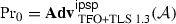.

This game is identical to Game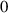except the challenger first guesses the target server S (and the associated time period) that accepts a spurious connection request and aborts if the guess is wrong. Since the probability of a correct guess is at least $$1/(|\mathcal {S}|+{q_{\mathsf {T}}})$$, we have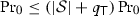.

This game replaces the PRF F used by S for TFO cookie generation with a truly random function f. By the PRF definition, it is straightforward to construct an efficient adversary $$\mathcal {B}$$ such that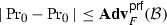.

Now, in Game, the TFO cookies generated by S are independent from each other for each client. We can bound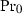by considering two cases. 1) $$\mathcal {A}$$ wins by sending a valid ACK packet in a full session. In this case, $$\mathcal {A}$$ must have generated a valid $$ack_C$$ by correctly guessing the target server’s TCP sequence number $$sqn_{S}$$. The winning probability of each guess is exactly $$1/2^{|{\mathtt {sqn}}|}$$. 2) $$\mathcal {A}$$ wins by sending a valid SYN packet in a resumption session. In this case, $$\mathcal {A}$$ must have forged a valid TFO cookie $$ck\in \{0,1\}^{64}$$. The winning probability of each forgery is exactly $$1/2^{|{\mathtt {ck}}|}$$ because the TFO cookie generation function is now a truly random function. By applying a union bound on the $${q_{\mathsf {S}}}$$ queries and noting that $$|{\mathtt {sqn}}|=32<64=|{\mathtt {ck}}|$$, we have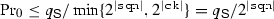. $$\square$$

### Remark

Note that the above second probability term is not very small since $$|{\mathtt {sqn}}|=32$$. Actually, an attacker can indeed successfully establish a TCP connection using a spoofed client IP address with roughly $$2^{32}$$ random guesses. However, our security bound is still acceptable because each guess made by the attacker corresponds to an “online” $${\mathsf {Send}}$$ query. Here, “online” means that the attacker has to interact with the server every time it makes a guess, no matter how many resources it may consume offline. Such online attacks are easy to detect and suppress.

KE Header Integrity. TFO+TLS 1.3 does not achieve this security notion because TCP headers are never authenticated. We find a new practical attack below, where an efficient adversary $$\mathcal {A}$$ can always get $$\mathbf {Adv}_\text {TFO+TLS 1.3}^{\mathsf {int\text {-}keh}}(\mathcal {A})=1$$:

TFO Cookie Removal. $$\mathcal {A}$$ can first make $$\pi _C^{i'}$$ complete a full handshake with $$\pi _S^{j'}$$ (via $${\mathsf {Connect}},{\mathsf {Send}}$$ queries), then query $${\mathsf {Resume}}(\pi _C^i,S,i')$$ ($$i'<i$$) to get the output packet $$(\text {IP}_C,\text {IP}_S,H,pd)$$, which is a SYN packet with a TFO cookie. $$\mathcal {A}$$ then modifies the $${\mathtt {opt}}$$ field of H to get a new $$H'\ne H$$ that contains no cookie. The resulting SYN packet will be accepted by a new server oracle $$\pi _S^j$$, which will then respond with a SYN-ACK packet that does not contain a TFO cookie, indicating a fallback to the standard 3-way TCP. As a result, a 1-RTT handshake is needed to complete the connection and any 0-RTT data sent with SYN would be retransmitted. This eliminates the entire benefit of TFO without being detected, resulting in reduced performance and increased handshake latency. A similar attack is possible by removing the TFO cookie in a server’s SYN-ACK packet.

Interestingly, clients are supposed to cache negative TFO responses and avoid sending TFO connections again for a lengthy period of time. This is because the most likely explanation for this behavior is that the server does not support TFO, but only standard TCP . As a result, performing this attack for a single connection prevents TFO from being used with this server for a lengthy time period (i.e., days or weeks).

KE Payload Integrity TFO+TLS 1.3 is secure in this regard simply because $${\mathtt {sid}}_\text {TLS}$$ consists of the payloads of all key exchange packets exchanged between the communicating parties before the client sets its session key. That is, for any client oracle that has a peer server oracle, by definition they observe the same $${\mathtt {sid}}_\text {TLS}$$ and hence no key exchange packet payload can be modified, i.e., $$\mathbf {Adv}_\text {TFO+TLS 1.3}^{\mathsf {int\text {-}kep}}(\mathcal {A})=0$$ for any efficient adversary $$\mathcal {A}$$.

SC Header Integrity TFO+TLS 1.3 does not achieve this security notion again because of the unauthenticated TCP headers.

A efficient adversary $$\mathcal {A}$$ can get $$\mathbf {Adv}_\text {TFO+TLS 1.3}^{\mathsf {int\text {-}h}}(\mathcal {A})=1$$ by either modifying the TCP header of an encrypted packet (e.g., reducing the $${\mathtt {window}}$$ value) or by forging a header-only packet (e.g., removing the payload of an encrypted packet and changing its $${\mathtt {ack}}$$ value). Such packets are valid and will be accepted by the receiving session oracle.

The above fact exposes the adversary’s ability to arbitrarily modify or even entirely forge the information in the TCP header, which is being relied on to provide reliable delivery, in-order delivery, flow control, and congestion control for the targeted flow. This leads to a whole host of availability attacks that the networking community has been slowly uncovering via manual investigation over the last 30 years [3, 16, 31, 32, 38,39,40, 44, 45, 52, 57, 58, 63, 70]. Some of the practical attacks are describedas follows:

TCP Flow Control Manipulation. An adversary with access to the communication channel can impact TCP’s flow control mechanism to decrease the sending rate or stall the connection by modifying TCP’s $${\mathtt {window}}$$ header field. This field controls the amount of received data the sender of this packet is prepared to buffer. By reducing this quantity, the throughput of the connection can be reduced and if it is set to zero the connection will completely stall.

One example of this attack would be to modify the window field to zero in a TCP packet containing a TLS-encrypted HTTP request. Since TCP headers are not authenticated, this modification will not be detected. As a result, when the server receives this request and attempts to send the response, it will believe that the client cannot currently accept any data and will delay sending the response. After some timeout, TCP will probe the client with a single packet of data to determine whether the window is still zero. If the adversary also modifies the responses to these probes, the connection will remain stalled indefinitely; otherwise, the connection will eventually recover after a lengthy delay.

TCP Acknowledgment Injection. An adversary who can observe a target connection and forge packets can inject new acknowledgment packets into the TCP connection. Acknowledgment packets have no data making them undetectable by either TLS or the application. However, they are used by congestion control to determine the allowed sending rate of a connection.

Injecting duplicate or very slowly increasing acknowledgments can be used to slow a target connection down drastically.  demonstrated a 12x reduction in throughput using this approach with the attacker required to expend only 40Kbps. This, of course, represents a significant performance degradation for a TFO+TLS 1.3 connection.

Injecting acknowledgments can also be used to dramatically increase the sending rate of a connection, turning it into a firehose that an attacker can point at their desired target. This is done by sending acknowledgments for data that has not been received yet, an attack known as Optimistic Ack . This attack renders TCP insensitive to congestion and can completely starve competing flows. It could be used with great effect to cause denial of service against a server or the Internet infrastructure as a whole .

Reset Authentication TFO+TLS 1.3 is insecure in this sense because its reset packet, TCP Reset, is an unauthenticated header-only packet. This leads to a practical attack below, where an efficient adversary $$\mathcal {A}$$ always gets $$\mathbf {Adv}_\text {TFO+TLS 1.3}^{\mathsf {rst\text {-}auth}}(\mathcal {A})=1$$:

TCP Reset Attack. $$\mathcal {A}$$ can first make two session oracles complete a handshake using $${\mathsf {Connect}},{\mathsf {Send}}$$ queries, then use $${\mathsf {Pack}},{\mathsf {Deliver}}$$ queries to let them exchange secure channel packets. By observing these packet headers, $$\mathcal {A}$$ can easily forge a valid reset packet by setting its RST bit to 1 and the remaining header fields to reasonable values. This attack will cause TCP to tear down the connection immediately without waiting for all data to be delivered.

Note that even an off-path adversary who can only inject packets into the communication channel may be able to accomplish this attack. The injected TCP reset packet needs to be within the receive window for the client or server, but  demonstrated that a surprisingly small number of packets is needed to achieve this, thanks to the large receive windows typically used by implementations.

### 5.2 QUIC over UDP

(1) Protocol Description

Referring to msACCE protocol syntax, the UDP+QUIC 1-RTT full handshake (see Fig. 4a) is a 2-stage msACCE protocol in the full mode and the UDP+QUIC 0-RTT resumption handshake (see Fig. 4b) is a 2-stage msACCE protocol in the resumption mode. The initial keys are set after sending or receiving ClientHello, and the final keys (i.e., session keys) are set after sending or receiving ServerHello.

According to the UDP and QUIC specifications [20, 46, 55], the UDP+QUIC header contains the UDP header (see Fig. 3 ) and the QUIC header (described below). As with the TCP header, we ignore the port numbers and checksum in the UDP header. Similarly, we also ignore the UDP length field because it affects only the length of the QUIC header and payload, while the adversary is already allowed to directly modify the packet length. We thus can completely omit the UDP header and define the header space $$\mathcal {H}$$ as containing the following QUIC header fields: an 8-bit public flag $${\mathtt {flag}}$$, a 64-bit connection ID $${\mathtt {cid}}$$, a 64-bit packet number $${\mathtt {pn}}$$, and other optional fields. Note that in reality QUIC header contains only, say 32, lower bits of $${\mathtt {pn}}$$, but this is enough to deduce the correct 64-bit value since the QUIC packet numbers are consecutive and start from 1. For simplicity, we consider a full 64-bit $${\mathtt {pn}}$$ in the header as with the previous work . We further define reset packets as those with the PUBLIC_FLAG_RESET bit (i.e., the 7-th bit of $${\mathtt {flag}}$$) set to 1. The header of a reset packet contains only $${\mathtt {flag}}$$ and $${\mathtt {cid}}$$.

In “Appendix A,” we formalize QUIC’s stateful AEAD scheme $$\mathsf {sAEAD}_\text {QUIC}$$ based on its underlying nonce-based AEAD scheme (instantiated with AES-GCM ). The associated data space $$\mathcal {AD}$$ contains the entire QUIC header. As with TLS 1.3, for UDP+QUIC we define $$\mathcal {M}_{\mathsf {KE}}$$ and $$\mathcal {M}_{\mathsf {SC}}$$ as consisting of bit strings differing in their first bits. $$\mathcal {M}_{\mathsf {pRST}}=\varnothing$$.

We refer to  for the detailed descriptions of $$\mathsf {scfg\_gen}$$ and QUIC handshake messages and key generations.

(2) Security Results

UDP+QUIC’s session identifier $${\mathtt {sid}}_\text {QUIC}$$ is defined as the ClientHello payload and ServerHello, excluding IP addresses. The msACCE-std security of UDP+QUIC follows from prior works . It has been proven in  that QUIC is QACCE-secure in the random oracle model based on the unforgeability of the signature scheme, computational Diffie–Hellman assumption , and nonce-based AEAD security. Note that msACCE-std with $$\mathsf {sAEAD}_\text {QUIC}$$ is semantically equivalent to QACCE with nonce-based $$\mathsf {AEAD}$$ and $${\mathsf {get\_iv}}$$ (defined in ), except that our model formalizes the advancement of time periods that was assumed by QACCE. More precisely, our model captures server-side local time periods (without time synchronization) while QACCE assumed a global time notion, but this difference does not affect the security results because QACCE essentially does not rely on time synchronization either. In other words, the same proofs in  can also show that QUIC is secure in the modified QACCE model that instead uses local time counters. As a result, their proofs can be easily adapted to show that UDP+QUIC achieves Server Authentication and level-1 Channel Security in our msACCE-std model. Note that one can also prove UDP+QUIC’s msACCE-std security by relying on the level-1 AEAD security of $$\mathsf {sAEAD}_\text {QUIC}$$ instead of the underlying nonce-based AEAD security, where the former can be reduced to the latter as shown in “Appendix A.” Note also that UDP+QUIC only achieves level-1 Channel Security (based on the level-1 AEAD security of $$\mathsf {sAEAD}_\text {QUIC}$$ ), nevertheless, as discussed in , QUIC implicitly prevents packet dropping and reordering by authenticating $${\mathtt {pn}}$$ in the packet header. It also prevents replays with frame sequence numbers encrypted in the payload. Therefore, UDP+QUIC essentially achieves level-4 authentication as TLS 1.3 does.

The msACCE-pauth security analyses are shown as follows.

IP-Spoofing Prevention In , QUIC has been proven secure against IP spoofing based on the AEAD security. Their IP-spoofing security notion is the same as our IP-Spoofing Prevention notion for UDP+QUIC except that ours additionally captures attacks in full sessions. However, since source-address tokens are validated in both full and resumption sessions, their results can be trivially adapted to show that UDP+QUIC achieves IP-Spoofing Prevention.

KE Header and Payload Integrity UDP+QUIC does not achieve these security notions because its first-round key exchange messages, i.e., InchoateClientHello and ServerReject, and any invalid ClientHello are not fully authenticated. Interestingly, a variety of existing attacks on QUIC’s availability discovered in  are all examples of key exchange packet manipulations (e.g., the server config replay attack, connection ID manipulation attack, etc.), but these attacks cause connection failure and hence are easy to detect. However, successful attacks breaking KE Header or Payload Integrity will be harder (if not impossible) to detect.

For KE Header Integrity, we do not find any harmful attacks but theoretical attacks exist. For instance, an efficient adversary $$\mathcal {A}$$ can get $$\mathbf {Adv}_\text {UDP+QUIC}^{\mathsf {int\text {-}keh}}(\mathcal {A})=1$$ as follows. $$\mathcal {A}$$ can first query $${\mathsf {Connect}}(\pi _C^i,S)$$ to get the output packet $$(\text {IP}_C,\text {IP}_S,H,pd)$$, then modify the $${\mathtt {flag}}$$ and $${\mathtt {pn}}$$ fields of H to get a new header $$H'\ne H$$ that only changes $${\mathtt {pn}}$$’s length but not its underlying value. The resulting packet will be accepted by a new server oracle $$\pi _S^j$$.

This attack has no practical impact on UDP+QUIC but it successfully modifies the protocol header without being detected.

For KE Payload Integrity, we find a new practical attack described below where an efficient adversary $$\mathcal {A}$$ can get $$\mathbf {Adv}_\text {UDP+QUIC}^{\mathsf {int\text {-}kep}}(\mathcal {A})\approx 1$$:

ServerReject Triggering. $$\mathcal {A}$$ can first let $$\pi _C^{i'}$$ complete a full handshake with $$\pi _S^{j'}$$ with $${\mathsf {Connect}},{\mathsf {Send}}$$ queries and then query $${\mathsf {Resume}}(\pi _C^i,S,i')$$ ($$i'<i$$) to get the output ClientHello packet. $$\mathcal {A}$$ then modifies its payload by replacing the source-address token stk with a random value, which with high probability is invalid. Sending this modified packet to a new server oracle $$\pi _S^j$$ will trigger a ServerReject packet containing a new valid $${\mathtt {stk}}$$. This as a result downgrades the original 0-RTT resumption connection to a full 1-RTT connection, which causes increased latency and results in the retransmission of any 0-RTT data. Note that this attack is hard to detect because $$\pi _C^i$$ may think its original $${\mathtt {stk}}'$$ has expired (although this does not happen frequently).

SC Header Integrity UDP+QUIC is secure in this regard because it does not allow header-only packets to be sent in the secure channel phases and the entire protocol header is taken as the associated data authenticated by the underlying encryption scheme. Therefore, UDP+QUIC’s SC Header Integrity can be reduced to its level-1 Channel Security. Formally, for any efficient adversary $$\mathcal {A}$$ there exists an efficient adversary B such that $$\mathbf {Adv}_\text {UDP+QUIC}^{\mathsf {int\text {-}h}}(\mathcal {A})\le \mathbf {Adv}_\text {UDP+QUIC}^{{\mathsf {cs}}\text {-}1}(\mathcal {B})$$. This is because $$\mathcal {B}$$ can simulate $$\mathcal {A}$$’s security experiment perfectly by answering the $${\mathsf {Pack}}$$ queries using $${\mathsf {Encrypt}}$$ with same inputs and forwarding the $${\mathsf {Deliver}}$$ queries to $${\mathsf {Decrypt}}$$, and if $$\mathcal {A}$$ outputs (Pi) and wins then $$\mathcal {B}$$ will also win by getting the secret bit $$b_P^{i}$$ from $${\mathsf {Decrypt}}$$.

Reset Authentication UDP+QUIC does not achieve this security notion because, similar to TCP Reset, its reset packet PublicReset is not authenticated either. In the following availability attack, an efficient adversary $$\mathcal {A}$$ can always get $$\mathbf {Adv}_\text {UDP+QUIC}^{\mathsf {rst\text {-}auth}}(\mathcal {A})=1$$:

PublicReset Attack. $$\mathcal {A}$$ can first make two session oracles complete a handshake using $${\mathsf {Connect}},{\mathsf {Send}}$$ queries, then use $${\mathsf {Pack}},{\mathsf {Deliver}}$$ queries to let them exchange secure channel packets. By observing these packet headers, $$\mathcal {A}$$ can easily forge a valid (plaintext) reset packet by setting its PUBLIC_FLAG_RESET bit to 1 and the remaining packet fields to reasonable values (which is easy because it simply contains the connection ID $${\mathtt {cid}}$$, the sequence number of the rejected packet, and a nonce to prevent replay). This attack will cause similar effects as described in the TCP Reset attack. Note that this vulnerability is fixed in QUIC[TLS] shown below.

### 5.3 QUIC[TLS] over UDP

(1) Protocol Description

As mentioned in the Background, QUIC[TLS] replaces QUIC’s key exchange with the TLS 1.3 key exchange, i.e., the stage keys are set in the same way as TLS 1.3. Therefore, the UDP+QUIC[TLS] 2-RTT full handshake is a 2-stage msACCE protocol in the full mode and the UDP+QUIC[TLS] 0-RTT resumption handshake is a 3-stage msACCE protocol in the resumption mode.

The UDP+QUIC[TLS] header is similar to the UDP+QUIC header and we can likewise omit the entire UDP header for our analysis. The detailed description of the QUIC[TLS] header is omitted here and referred to . A reset packet in UDP+QUIC[TLS] looks indistinguishable from a non-reset secure channel packet, but accepting it leads to a session reset.

The stateful AEAD scheme $$\mathsf {sAEAD}_\text {QUIC[TLS]}$$ used by QUIC[TLS] to encrypt packets is very similar to $$\mathsf {sAEAD}_\text {QUIC}$$, except that the nonce of the underlying nonce-based AEAD is computed as the exclusive OR of the secret iv (part of the key generated in $$\mathsf {sAEAD}_\text {QUIC[TLS]}$$) and the packet number. As with $$\mathsf {sAEAD}_\text {QUIC}$$, the level-1 AEAD security of $$\mathsf {sAEAD}_\text {QUIC[TLS]}$$ is reduced to the security of its underlying nonce-based AEAD, following a proof very similar to that of Theorem 3 in “Appendix A.” The associated data space $$\mathcal {AD}$$ contains the entire QUIC[TLS] header.

Note that QUIC[TLS] applies a header protection mechanism on the encrypted packet to further hide some header fields (e.g., those related to the packet number), which provides stronger nonce-hiding security (a notion proposed by ). For simplicity, we do not consider this new feature and assume header protection will not weaken the security of the encrypted packet, as our analysis focuses on the packet header’s integrity rather than its confidentiality. We refer to  for a mechanized analysis of QUIC[TLS]’s record layer security that covers header protection.

QUIC[TLS] enforces different frame types for encrypted key exchange, secure channel, and pre-reset messages. For simplicity, we define $$\mathcal {M}_{\mathsf {KE}},\mathcal {M}_{\mathsf {SC}},\mathcal {M}_{\mathsf {pRST}}$$ as consisting of bit strings differing in their first two bits. For UDP+QUIC[TLS], $$\mathsf {scfg\_gen}$$ is undefined.

QUIC[TLS] also provides address validation with a secure token generated by the server, similar to the case in Google’s QUIC. We discuss QUIC[TLS]’s stateless reset mechanism later in the security analysis of Reset Authentication and refer to [36, 68] for the detailed UDP+QUIC[TLS] handshake messages and key generations.

(2) Security Results

UDP+QUIC[TLS]’s session identifier $${\mathtt {sid}}_\text {QUIC[TLS]}$$ is defined as all key exchange messages (that could be encrypted) from ClientHello to ServerFinished, excluding the header fields. By construction, UDP+QUIC[TLS] inherits the msACCE-std security from TLS 1.3, but uses $$\mathsf {sAEAD}_\text {QUIC[TLS]}$$ for encryption. That is, it achieves level-1 Channel Security and implicitly achieves level-4 authentication in the same way as UDP+QUIC. The IP-Spoofing Prevention of UDP+QUIC[TLS] follows a address validation scheme very similar to UDP+QUIC. In particular, if the token is generated with an authenticated encryption scheme, then the IP-Spoofing Prevention security is reduced to the encryption scheme’s authenticity security, as with UDP+QUIC. However, such an address validation scheme suffers from the same kind of availability attack against KE Payload Integrity as ServerReject Triggering for UDP+QUIC, where the adversary replaces the address-validation token with a random value to downgrade a 0-RTT resumption connection. As noted in , an adversary can also modify the unauthenticated ACK frames in the Initial packets without being detected. Furthermore, UDP+QUIC[TLS] achieves SC Header Integrity in the same way as UDP+QUIC. We are only left to show its security of KE Header Integrity and Reset Authentication.

KE Header Integrity UDP+QUIC[TLS] does not achieve these security notions because its first-round Initial packets (see ) are not authenticated. For instance, an efficient adversary $$\mathcal {A}$$ can get $$\mathbf {Adv}_\text {UDP+QUIC[TLS]}^{\mathsf {int\text {-}keh}}(\mathcal {A})=1$$ as follows. $$\mathcal {A}$$ first queries $${\mathsf {Connect}}(\pi _C^i,S)$$ to get $$\pi _C^i$$’s Initial packet $$(\text {IP}_C,\text {IP}_S,H,pd)$$. Then, as described in , $$\mathcal {A}$$ can decrypt this packet with its destination connection ID cid in H, change it to another value $$cid'$$, and re-encrypt the whole packet with this new $$cid'$$. The resulting packet $$(\text {IP}_C,\text {IP}_S,H',pd')$$, where $$H\ne H'$$, is valid and will be accepted by a new server oracle $$\pi _S^j$$ without being detected by the client. However, this is only a theoretical attack with no practical impact.

Reset Authentication In UDP+QUIC[TLS], the stateless reset works as follows. One party generates a 128-bit reset token using its static key and a random 64-bit QUIC connection ID as input. Then, this token (carried within the pre-reset message) is sent to the other party in a secure channel phase. Later, the same party that generated this token can perform a stateless reset by regenerating the token and sending it to the other party in clear (via a reset packet).

The Reset Authentication security of UDP+QUIC[TLS] can be reduced to its level-1 Channel Security and the PRF security of the reset token generation function F (which can be instantiated with an HMAC  for instance), as shown in the following theorem:

### Theorem 2

For any efficient adversary $$\mathcal {A}$$ making at most $${q_{\mathsf {D}}}$$ $${\mathsf {Deliver}}$$ queries, there exist efficient adversaries $$\mathcal {B}$$ and $$\mathcal {C}$$ such that:

\begin{aligned} {\mathbf{Adv}}_{\mathrm{UDP+QUIC[TLS]}}^{{\mathsf {rst}}\text {-}{\mathsf {auth}}}({\mathcal {A}})\le & {} | {\mathcal {P}}|{\mathbf{Adv}}_{F}^{{\mathsf {prf}}}({\mathcal {B}})+|{\mathcal {P}}|{\mathbf{Adv}} _{\mathrm{UDP+QUIC[TLS]}}^{{{\mathsf {cs}}\text {-}1}}({\mathcal {C}})+\frac{|{\mathcal {P}}|{q_{\mathsf {D}}}}{2^{|{\texttt {rtk}}|}}\\&+\frac{|{\mathcal {P}}|N^2}{2^{|{\texttt {cid}}|}}, \end{aligned}

where $$|\mathcal {P}|$$ is the number of parties, N is the maximum number of sessions for each party, $$|{\mathtt {rtk}}|$$ is the bit length of a reset token, and $$|{\mathtt {cid}}|$$ is the bit length of a connection ID.

### Proof

Consider a sequence of games (i.e., experiments) and let $$\Pr _i,i\ge 0$$ denote the winning probability of $$\mathcal {A}$$ in Game i.

This is the original Reset Authentication experiment, so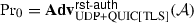.

This game is the same as Gameexcept it aborts if the connection IDs repeat for a party. Since the probability of such a collision for each party is at most $$N^2/2^{|{\mathtt {cid}}|}$$, by a union bound we have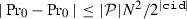.

The challenger proceeds as before except it first guesses the target party P (recall that $$\mathcal {A}$$ outputs (Pi) in the end) and aborts if the guess is wrong. Since the probability of a correct guess is at least $$1/|\mathcal {P}|$$, we have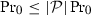.

This game replaces the PRF F used by P for reset token generation with a truly random function f. By the PRF definition, it is straightforward to construct an efficient adversary $$\mathcal {B}$$ such that.

This game replaces the pre-reset packet (if any) output by the target session oracle $$\pi _P^i$$ (i.e., output by $${\mathsf {Pack}}(\pi _P^i,\cdot ,{\mathtt {prst}})$$) with encryption of an arbitrarily fixed pre-reset message $$m^*_{\mathsf {prst}}\in \mathcal {M}_{\mathsf {pRST}}$$. As illustrated below, there exists an efficient adversary $$\mathcal {C}$$ against the level-1 Channel Security of UDP+QUIC[TLS] such that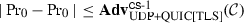.

$$\mathcal {C}$$ samples the static reset token generation keys for all parties and simulate $${\mathsf {Pack}}$$ and $${\mathsf {Deliver}}$$ queries with its $${\mathsf {Encrypt}}$$ and $${\mathsf {Decrypt}}$$ queries. In particular, for a $${\mathsf {Pack}}(\cdot ,\cdot ,{\mathtt {prst}})$$ query made to a session oracle of some party other than the target party P, $$\mathcal {C}$$ generates the reset token with $$F_k(cid)=rtk$$ (where k is the token generation key of the specified party and cid is the connection ID used by the specified session oracle) and forms the pre-reset message $$m_{\mathsf {prst}}$$ that carries that token $$F_k(cid)$$, then queries $${\mathsf {Encrypt}}$$ with $$(m_{\mathsf {prst}},m_{\mathsf {prst}})$$ as the challenge message pair and uses the output ciphertext to form a pre-reset packet sent to $$\mathcal {A}$$. For a $${\mathsf {Pack}}(\cdot ,\cdot ,{\mathtt {prst}})$$ query made to a session oracle of the target party P but not the target session i, the token is instead generated with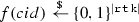via lazy sampling and the rest is the same. For a $${\mathsf {Pack}}(\pi _P^i,\cdot ,{\mathtt {prst}})$$ query (i.e., to the target session oracle), $$\mathcal {C}$$ generates a random reset token f(cid) (if f(cid) is not yet set) and forms a pre-reset message $$m_{\mathsf {prst}}$$ that carries that token f(cid), then queries $${\mathsf {Encrypt}}$$ with $$(m_{\mathsf {prst}},m^*_{\mathsf {prst}})$$ as the challenge message pair and uses the output ciphertext to form a pre-reset packet sent to $$\mathcal {A}$$. The $${\mathsf {Pack}}(\cdot ,\cdot ,{\mathtt {rst}})$$ queries can be simulated in a similar way, except that no $${\mathsf {Encrypt}}$$ query is needed and the reset token is used to form a reset packet sent to $$\mathcal {A}$$. When receiving a reset packet via a $${\mathsf {Deliver}}$$ query, $$\mathcal {C}$$ checks if the reset token carried in this packet matches the previously received reset token carried in the pre-reset packet and then accepts it if and only if the check passes. It is not hard to see that $$\mathcal {C}$$ can perfectly simulate Gamein the left world ($$b_P^i=0$$) or Gamein the right world ($$b_P^i=1$$). $$\mathcal {C}$$ outputs 1 if and only if $$\mathcal {A}$$ wins.

Now, in Game, the random pre-reset message of the target $$\pi _P^i$$ is independent from $$\mathcal {A}$$’s view and each guess is correct with probability $$1/2^{|{\mathtt {rtk}}|}$$. By a union bound, we have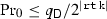. $$\square$$

### Remark

Recall that $$|{\mathtt {rtk}}|=128$$ and $$|{\mathtt {cid}}|=64$$. Similar to the remark following Theorem 1, regarding the last probability term, any attacker has to observe roughly $$2^{32}$$ online sessions in order to find a connection ID collision. To forge (basically replay) a valid reset packet, the attacker has to make roughly $$2^{32}$$ online $${\mathsf {Pack}}(\cdot , \cdot , {\mathtt {rst}})$$ queries to the target party to keep record of the reset packets for those observed sessions since each session is typically alive for a short time; this is an online attack that is easy to detect and suppress. Note that in theory if the target party can open $$2^{32}$$ concurrent sessions, then the attacker only needs to make a single online query; however, in practice this is very unlikely since there are only $$2^{16}-1$$ TCP sockets per IP address. Note also that the attacker does not get to choose which session to be maliciously reset since a collision can occur at any time.

## 6 Conclusion

Our work is the first to provide a thorough, formal, and fine-grained security comparison of the most efficient secure channel establishment protocols on the market today. By including packet-level attacks in our analysis, our results shed light on how the reliability, flow control, and congestion control of TFO+TLS 1.3, UDP+QUIC, and UDP+QUIC[TLS] compare besides their basic security, in adversarial settings.

We show that availability functionalities provided by transport-layer protocols like TCP can be compromised due to lack of packet-level authentication, which may undermine the performance of their supporting application-layer protocols. To protect against availability attacks, secure channel establishment protocols should better implement and authenticate their own transport functionalities like QUIC does. Besides, the key exchange packet integrity should also be scrutinized to avoid serious undetectable availability attacks.

We hope that our models will help protocol designers in their future protocol analyses and that our results will help practitioners better understand the advantages and limitations of secure channel establishment protocols.